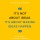Home
IT Knowledge
Inspiration
Languages
EN

# Bash - measure command execution time in milliseconds

9 points
Created by:marc_so
264

In this short article, we would like to show how in Bash, measure command execution duration in milliseconds.

Quick solution:

``t1=\$(date +%s%3N); some_command_here; t2=\$(date +%s%3N); echo "\$((t2-t1)) ms"``

## Practical example

In the below example the command line sleeps 3 seconds (returns sleeping + execution time).

``t1=\$(date +%s%3N); sleep 3; t2=\$(date +%s%3N); echo "\$((t2-t1)) ms"``

Example output:

``3028 ms``